# Number System Conversion

As you know decimal, binary, octal and hexadecimal number systems are positional value number systems. To convert binary, octal and hexadecimal to decimal number, we just need to add the product of each digit with its positional value. Here we are going to learn other conversion among these number systems.

## Decimal to Binary

Decimal numbers can be converted to binary by repeated division of the number by 2 while recording the remainder. Let’s take an example to see how this happens.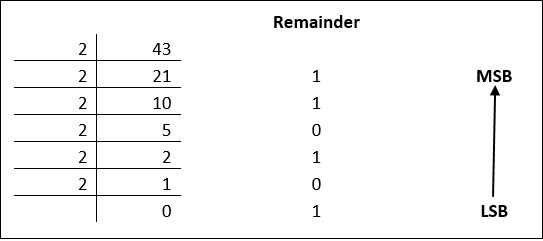The remainders are to be read from bottom to top to obtain the binary equivalent.

4310 = 1010112

## Decimal to Octal

Decimal numbers can be converted to octal by repeated division of the number by 8 while recording the remainder. Let’s take an example to see how this happens.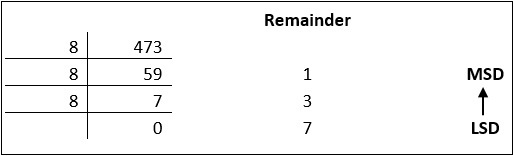Reading the remainders from bottom to top,

47310 = 7318

Decimal numbers can be converted to octal by repeated division of the number by 16 while recording the remainder. Let’s take an example to see how this happens.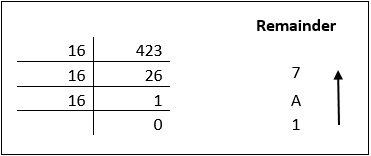Reading the remainders from bottom to top we get,

42310 = 1A716

## Binary to Octal and Vice Versa

To convert a binary number to octal number, these steps are followed −

• Starting from the least significant bit, make groups of three bits.

• If there are one or two bits less in making the groups, 0s can be added after the most significant bit

• Convert each group into its equivalent octal number

Let’s take an example to understand this.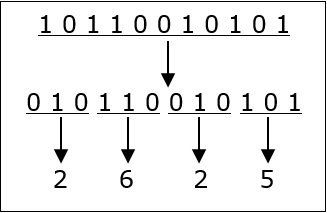101100101012 = 26258

To convert an octal number to binary, each octal digit is converted to its 3-bit binary equivalent according to this table.

 Octal Digit 0 1 2 3 4 5 6 7 Binary Equivalent 000 001 010 011 100 101 110 111

546738 = 1011001101110112

To convert a binary number to hexadecimal number, these steps are followed −

• Starting from the least significant bit, make groups of four bits.

• If there are one or two bits less in making the groups, 0s can be added after the most significant bit.

• Convert each group into its equivalent octal number.

Let’s take an example to understand this.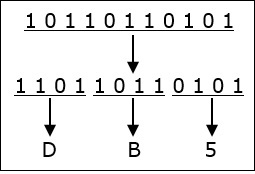101101101012 = DB516

To convert an octal number to binary, each octal digit is converted to its 3-bit binary equivalent.